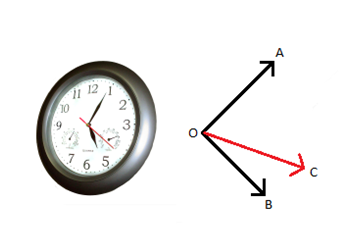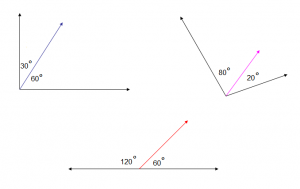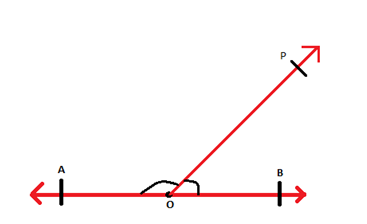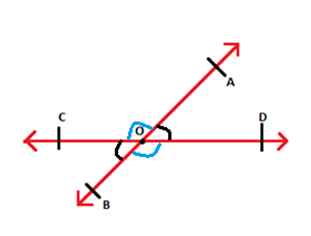Courses

Adjacent Angles & Vertical Opposite Angles Notes - Class 6

Class 6: Adjacent Angles & Vertical Opposite Angles Notes - Class 6

The document Adjacent Angles & Vertical Opposite Angles Notes - Class 6 is a part of Class 6 category.
All you need of Class 6 at this link: Class 6

What are opposite angles and adjacent?

December 3, 20171 Comment

The measure of rotation of a ray, when it is rotated about its end point is known as the angle formed by the ray between its initial and final position.

Consider a wall clock as shown in the following fig. 1. The minute hand and second hand of clock form one angle represented as ∠AOC and the hour hand forms another angle with the second hand represented as∠COB. Both these pair of angles i.e.∠AOC and ∠COB lies next to each other and are known as adjacent angles.∠AOC and ∠COB have a common vertex, a common arm and the uncommon arms lie on either side of the common arms. Such angles are known as

Here are some examples of Adjacent angles:Linear Pair

Pair of adjacent angles whose measures add up to form a straight angle is known as a linear pair. The angles in a linear pair are supplementary.

Consider the following figure in which a ray OPOP→ stand on the line segment AB¯¯¯¯¯¯¯¯AB¯   as shown:The angles ∠POB and ∠POA are formed at O. ∠POB and ∠POA are adjacent angles and they are supplementary i.e. ∠POB + ∠POA = ∠AOB = 180°

∠POB and ∠POA are adjacent to each other and when the sum of adjacent angles is 180° then such angles form linear pair of angles.

Vertically Opposite Angles

When a pair of lines intersects, as shown in the fig. below, four angles are formed. ∠AOD and ∠COB are vertically opposite to each other and ∠AOC and ∠BOD are vertically opposite to each other. These angles are also known as vertical angles or opposite angles.Thus, when two lines intersect, two pair of vertically opposite angles are formed i.e. ∠AOD, ∠COB and ∠AOC, ∠BOD.

According to vertical angle theorem, in a pair of intersecting lines the vertically opposite angles are equal.

The document Adjacent Angles & Vertical Opposite Angles Notes - Class 6 is a part of Class 6 category.
All you need of Class 6 at this link: Class 6Use Code STAYHOME200 and get INR 200 additional OFF Use Coupon Code

Top Courses for Class 6Top Courses for Class 6Track your progress, build streaks, highlight & save important lessons and more!

,

,

,

,

,

,

,

,

,

,

,

,

,

,

,

,

,

,

,

,

,

;